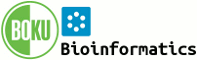University of Natural Resources and Life Sciences (BOKU) Institute of Computational Biology

The `%CALC{"formula"}%` macro is handled by the SpreadSheetPlugin. There are around 90 formulae, such as `\$ABS()`, `\$EXACT()`, `\$EXISTS()`, `\$GET()/\$SET()`, `\$IF()`, `\$LOG()`, `\$LOWER()`, `\$PERCENTILE()`, `\$TIME()`, `\$VALUE()`.
• `%CALC{"\$SUM(\$ABOVE())"}%` returns the sum of all cells above the current cell
• `%CALC{"\$EXISTS(Web.SomeTopic)"}%` returns `1` if the topic exists
• `%CALC{"\$UPPER(Collaboration)"}%` returns `COLLABORATION`